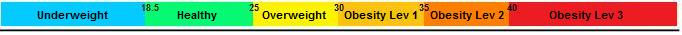BMI (Body Max Index)
The Body Mass Index (BMI) expresses the ratio between the weight and height.It is a mathematical formula for the weight of a person is divided by your height squared.The BMI value is more closely connected to body fat than any otherindicators of weight and height. Individuals with a BMI between 25 and 29.9 are considered overweight,while individuals with a BMI equal to or greater than 30 are considered obese. In any case, BMI,as the same weight are not the best indicators of health. For example, an athlete in goodmuscular conditions may have a high BMI because of the density of muscle mass, reason why we calculate the percentage of body fat through skinfold.A person with rather low BMI might be overweight.### BMI calculator

#### Instruction:

Enter all data in the appropriate fields and press calculate
 Sex : Male Female Height (cm): Weight (kg): BMI (%):